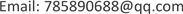1. 引言

2. 工程概况以及模型的建立

3. 计算结果的分析和讨论

YJK model main calculation result

T2 = 3.2030(Y)T5 = 0.8197T2 = 3.2034(Y)T5 = 0.8195
T3 = 2.3997(T)T6 = 0.6886T3 = 2.3993(T)T6 = 0.6884

T2 = 3.1975(Y)T5 = 0.8175T2 = 3.0789(Y)T5 = 0.8018
T3 = 2.3937(T)T6=0.6868T3 = 2.3936(T)T6 = 0.6717

V ≤ 1 γ R E [ 1 λ − 0.5 ( 0.4 f t b w h w 0 + 0.1 N A w A ) + 0.8 f y h A s h s h w 0 ] (1)

A——剪力墙全截面积；

Aw——T形或I形截面剪力墙腹板的面积，矩形截面时应取A；

Ash——为在水平分布筋间距内的水平分布筋面积(cm2)；

s——剪力墙水平分布钢筋间距；

ft——混凝土轴心抗位强度设计值；

fyh——剪力墙水平分布钢筋的抗位强度设计值。

《高规》7.2.6对地震设计状况下剪力墙墙肢截面剪力设计值的规定：

V ≤ 1 γ R E ( 0.20 β c f c b w h w 0 ) (2)

V ≤ 1 γ R E ( 0.15 β c f c b w h w 0 ) (3)

λ = M C / ( V C h w 0 ) (4)

hw0——剪力墙截面有多高效， h w 0 = h w − a ′ s ；

βc——混凝土强度影响系数，按《高层建筑混凝土技术规程》第6.2.6条采用；

λ——剪跨比，其中MC、VC应取同一组合、未经调整的墙肢截面弯矩、剪力计算值，并取墙肢上、下端截面计算剪跨比的较大值；

fc——混凝土轴心抗压强度设计值；

γRE——承载力抗震调整系数，按《混凝土结构技术规范》第11.1.6条采用；

a ′ s ——剪力墙受压区端部钢筋合力点到受压区边缘的距离。

4. 结论

1) 地下室顶板错层对整体结构的自振特性、内力及变形特征影响很小；地下室顶板具备作为上部结构的嵌固部位的条件。

2) 采用斜梁板构造措施能有效降低水平地震作用下错层处剪力墙的剪力，且室内外交界处的剪力墙应力分布较为均匀，没有产生应力突变。

3) 采用分步错层能够降低剪力墙的剪力，应力分布也较为均匀，但错层处剪力墙的最大剪力值和应力峰值均大于斜梁板措施。

4) 采用加腋构造措施对剪力墙剪力的影响程度较小，应力分布与错层无加强措施的剪力墙基本一致，且存在明显的应力集中，但错层处剪力墙的最大剪力值和应力峰值均有不同程度的降低。